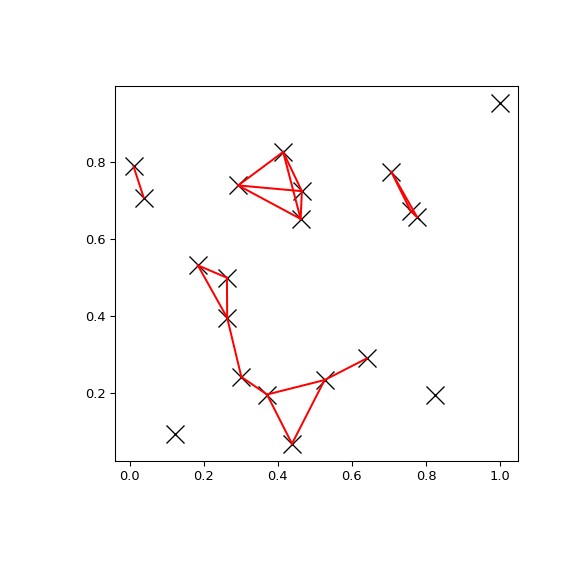# scipy.spatial.KDTree.query_pairs¶

KDTree.query_pairs(r, p=2.0, eps=0, output_type='set')[source]

Find all pairs of points in self whose distance is at most r.

Parameters
rpositive float

The maximum distance.

pfloat, optional

Which Minkowski norm to use. p has to meet the condition `1 <= p <= infinity`.

epsfloat, optional

Approximate search. Branches of the tree are not explored if their nearest points are further than `r/(1+eps)`, and branches are added in bulk if their furthest points are nearer than `r * (1+eps)`. eps has to be non-negative.

output_typestring, optional

Choose the output container, ‘set’ or ‘ndarray’. Default: ‘set’

New in version 1.6.0.

Returns
resultsset or ndarray

Set of pairs `(i,j)`, with `i < j`, for which the corresponding positions are close. If output_type is ‘ndarray’, an ndarry is returned instead of a set.

Examples

You can search all pairs of points in a kd-tree within a distance:

```>>> import matplotlib.pyplot as plt
>>> import numpy as np
>>> from scipy.spatial import KDTree
>>> rng = np.random.default_rng()
>>> points = rng.random((20, 2))
>>> plt.figure(figsize=(6, 6))
>>> plt.plot(points[:, 0], points[:, 1], "xk", markersize=14)
>>> kd_tree = KDTree(points)
>>> pairs = kd_tree.query_pairs(r=0.2)
>>> for (i, j) in pairs:
...     plt.plot([points[i, 0], points[j, 0]],
...             [points[i, 1], points[j, 1]], "-r")
>>> plt.show()
```